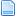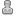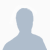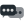Hello Guest it is November 18, 2019, 11:01:53 AM

###Author Topic: Edit post for xy position move then z  (Read 3194 times)

0 Members and 1 Guest are viewing this topic.

####Programinator•16##### Edit post for xy position move then z
« on: December 25, 2015, 05:33:43 PM »
This is what my gcode is coming out like:

G90G80G20G49
G0Z0.1000
G0 X-0.5662 Y0.9343 Z0.1000
G1   Z-0.1250 F30

I would like it to come out like this:

G90G80G20G49
G0 X-0.5662 Y0.9343
G0Z0.1000
G1   Z-0.1250 F30

This is what my post looks like:

;
START = "([FILENAME])"
START = "(Material Size) (X=[XSIZE], Y=[YSIZE], Z=[ZMATERIAL])"
START = "(Tool Number:[T]) ([TOOLDESC])"
START = "G90G80G20G49"
START = "G0[ZH]"
;
; Program moves
;
FIRST_RAPID_RATE_MOVE  = "G0 [X] [Y] [Z]"
;
FIRST_FEED_RATE_MOVE   = "G1 [X] [Y] [Z] [F]"
FEED_RATE_MOVE         = "[X] [Y] [Z]"
;
FIRST_CW_ARC_MOVE      = "G2 [X] [Y] [J] [F]"
CW_ARC_MOVE            = "G2 [X] [Y] [J]"
;
FIRST_CCW_ARC_MOVE     = "G3 [X] [Y] [J] [F]"
CCW_ARC_MOVE           = "G3 [X] [Y] [J]"
;
NEW_SEGMENT_POST_TOOLCHANGE= "(Tool Number:[T]) ([TOOLDESC])"
NEW_SEGMENT_POST_TOOLCHANGE= "M3 [S)"
;
; End of file
;
END = "G0[ZH]"
END = "G0[XH][YH]"
END = "M30"
; M30 = program end and rewind

I have tried a bunch of different codes and i cant get it to post like i wans

Im sure its an easy fix.  Any help would be appreciated

Thanks

« Last Edit: December 25, 2015, 05:35:54 PM by Programinator »

####BR549•6,916##### Re: Edit post for xy position move then z
« Reply #1 on: December 25, 2015, 07:43:30 PM »
Are you SURE you want to do it that way ??. Normally you would want to FIRST move to Z rapid height THEN move in XY to teh start position then lower Z at feedrate to start.

That is what your first example is doing.

IF you first MOVE in XY without lifting Z to rapid clearnace you could easily tear something up if you hit something with teh tool. The Machine has NO idea where anything is so that is teh reason you would move it to a safeZ height BEFORE teh XY moves.

What Cam program is that ?  I can guess what to change but easier and safer to just look it up then you are sure.

Just a thought, (;-) TP

####Programinator•16##### Re: Edit post for xy position move then z
« Reply #2 on: December 25, 2015, 08:28:58 PM »
Yes im sure this is what i want.  I always start my program with the tool above the part.  For my way of doing things this is way better.  Let me know if you have any ideas on how to change it and i can test it out.

Its for artcam

Thanks

####BR549•6,916##### Re: Edit post for xy position move then z
« Reply #3 on: December 25, 2015, 08:45:58 PM »
This is just a best guess , see what it does.

;
START = "([FILENAME])"
START = "(Material Size) (X=[XSIZE], Y=[YSIZE], Z=[ZMATERIAL])"
START = "(Tool Number:[T]) ([TOOLDESC])"
START = "G90G80G20G49"
;START = "G0[ZH]"
;
; Program moves
;
FIRST_RAPID_RATE_MOVE  = "G0 [X] [Y] "
RAPID_RATE_MOVE  = "G0 [X] [Y] [Z]"
;
FIRST_FEED_RATE_MOVE   = "G1 [X] [Y] [Z] [F]"
FEED_RATE_MOVE         = "[X] [Y] [Z]"
;
FIRST_CW_ARC_MOVE      = "G2 [X] [Y] [J] [F]"
CW_ARC_MOVE            = "G2 [X] [Y] [J]"
;
FIRST_CCW_ARC_MOVE     = "G3 [X] [Y] [J] [F]"
CCW_ARC_MOVE           = "G3 [X] [Y] [J]"
;
NEW_SEGMENT_POST_TOOLCHANGE= "(Tool Number:[T]) ([TOOLDESC])"
NEW_SEGMENT_POST_TOOLCHANGE= "M3 [S)"
;
; End of file
;
END = "G0[ZH]"
END = "G0[XH][YH]"
END = "M30"
; M30 = program end and rewind

####BR549•6,916##### Re: Edit post for xy position move then z
« Reply #4 on: December 25, 2015, 08:49:46 PM »
Artcam HAS a post configuration manual have you read it ??

(;-) TP

####ger21••6,288##### Re: Edit post for xy position move then z
« Reply #5 on: December 25, 2015, 08:59:40 PM »
Try this, but I'm with terry, you should leave it like it is.

;
START = "([FILENAME])"
START = "(Material Size) (X=[XSIZE], Y=[YSIZE], Z=[ZMATERIAL])"
START = "(Tool Number:[T]) ([TOOLDESC])"
START = "G90G80G20G49"
;
; Program moves
;
FIRST_RAPID_RATE_MOVE  = "G0 [X] [Y] [Z]"
FIRST_RAPID_RATE_MOVE  ="G0[ZH]"
Gerry

2010 Screenset
http://www.thecncwoodworker.com/2010.html

JointCAM Dovetail and Box Joint software
http://www.g-forcecnc.com/jointcam.html

####Programinator•16##### Re: Edit post for xy position move then z
« Reply #6 on: December 25, 2015, 09:42:33 PM »

;
START = "([FILENAME])"
START = "(Material Size) (X=[XSIZE], Y=[YSIZE], Z=[ZMATERIAL])"
START = "(Tool Number:[T]) ([TOOLDESC])"
START = "G90G80G20G49"
;START = "G0[ZH]"
;
; Program moves
;
FIRST_RAPID_RATE_MOVE  = "G0 [X] [Y] "
RAPID_RATE_MOVE  = "G0 [X] [Y] [Z]"

This code posts like this:  Not the result i need.  i will try the other suggestion now
THanks for trying

(text)
(Material Size) (X=39.370, Y=9.840, Z=1.500)
(Tool Number:1) (0.250 inches dia. slot drill)
G90G80G20G49
G0 X-0.5662 Y0.9343
G1   Z-0.1250 F30

####Programinator•16##### Re: Edit post for xy position move then z
« Reply #7 on: December 25, 2015, 09:45:38 PM »
The second suggestion fails to post.  "Duplicate statement found"

THanks for trying

any other suggestions

####ger21••6,288##### Re: Edit post for xy position move then z
« Reply #8 on: December 25, 2015, 09:56:51 PM »
Try this:

;
START = "([FILENAME])"
START = "(Material Size) (X=[XSIZE], Y=[YSIZE], Z=[ZMATERIAL])"
START = "(Tool Number:[T]) ([TOOLDESC])"
START = "G90G80G20G49"
;
; Program moves
;
FIRST_RAPID_RATE_MOVE  = "G0 [X] [Y] [Z]"
RAPID_RATE_MOVE  ="G0[ZH]"
Gerry

2010 Screenset
http://www.thecncwoodworker.com/2010.html

JointCAM Dovetail and Box Joint software
http://www.g-forcecnc.com/jointcam.html

####Programinator•16##### Re: Edit post for xy position move then z
« Reply #9 on: December 25, 2015, 11:25:54 PM »
Well this is what i ended up doing.  a machine coordanate move to z-.1 then xy to work offset move then z

I like it like this because i change tools manually off the part on the xy and if i had a move to z.1 first then xy it will hit clamps.

This way i dont have to worry about it.

I dont understand why the way you guys suggested will not work.  But this way will do the trick

;
START = "([FILENAME])"
START = "(Material Size) (X=[XSIZE], Y=[YSIZE], Z=[ZMATERIAL])"
START = "(Tool Number:[T]) ([TOOLDESC])"
START = "G90G80G20G49"
START = "G90G53Z-.1"
START = "G0[XH][YH]"
START = "G0[ZH]"

;
; Program moves
;
FIRST_RAPID_RATE_MOVE  = "G0 [X] [Y] [Z]"
« Last Edit: December 25, 2015, 11:27:56 PM by Programinator »